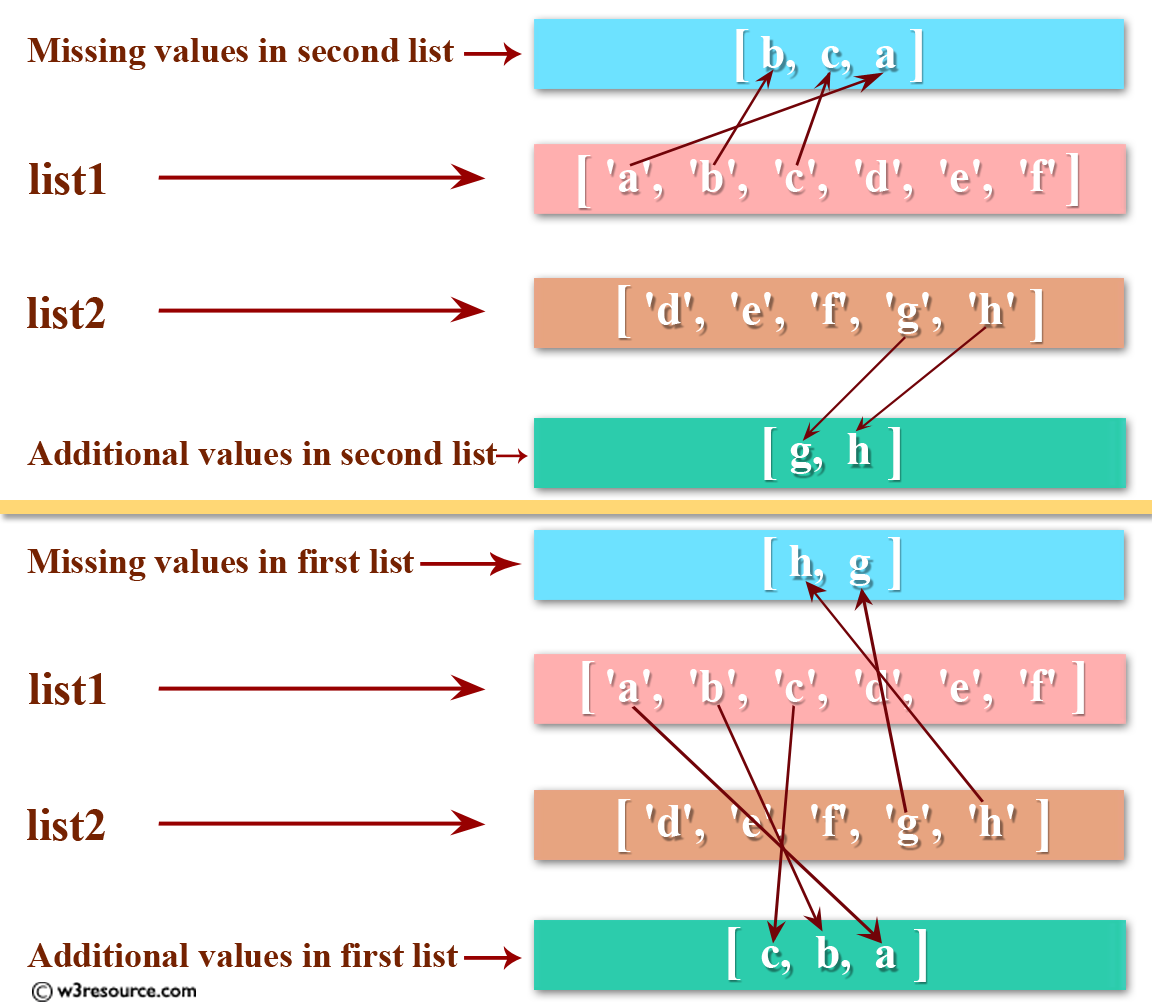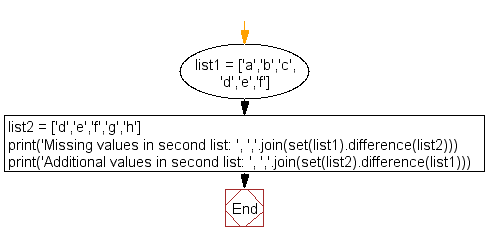﻿ Python: Find missing and additional values in two lists - w3resource# Python: Find missing and additional values in two lists

## Python List: Exercise - 42 with Solution

Write a Python program to find missing and additional values in two lists.Sample Solution :-

Python Code :

``````list1 = ['a','b','c','d','e','f']
list2 = ['d','e','f','g','h']
print('Missing values in second list: ', ','.join(set(list1).difference(list2)))
print('Additional values in second list: ', ','.join(set(list2).difference(list1)))
```
```

Sample Output:

```Missing values in second list:  b,c,a
Additional values in second list:  g,h
```

Flowchart:## Visualize Python code execution:

The following tool visualize what the computer is doing step-by-step as it executes the said program:

Python Code Editor:

Have another way to solve this solution? Contribute your code (and comments) through Disqus.

What is the difficulty level of this exercise?

Test your Python skills with w3resource's quiz

﻿

## Python: Tips of the Day

```print(2_000_000)
```2000000